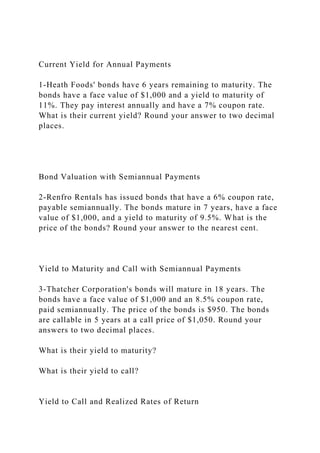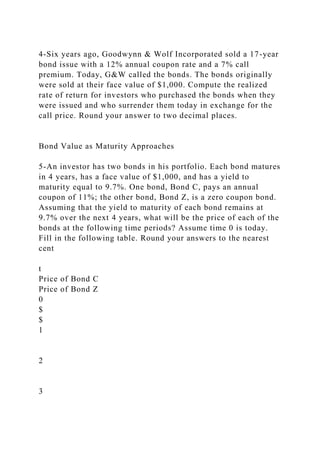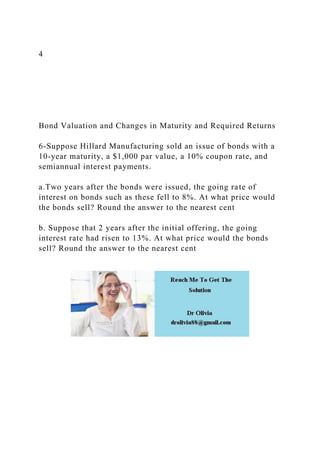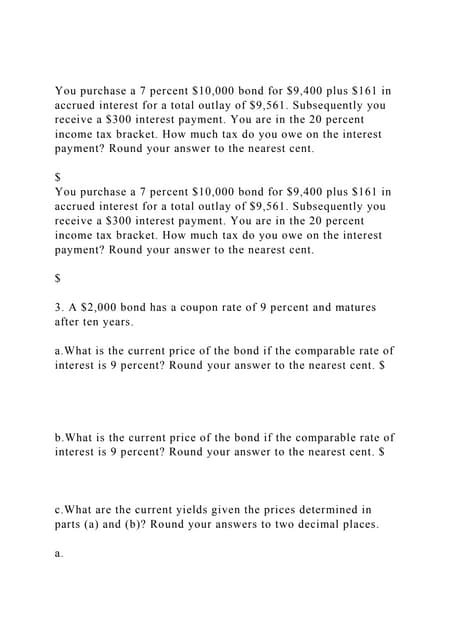PublicitéProchain SlideShareYou purchase a 7 percent \$10,000 bond for \$9,400 plus \$161 in accr.docx
Chargement dans ... 3
1 sur 3
Publicité

### Current Yield for Annual Payments1-Heath Foods bonds have 6 y.docx

1. Current Yield for Annual Payments 1-Heath Foods' bonds have 6 years remaining to maturity. The bonds have a face value of \$1,000 and a yield to maturity of 11%. They pay interest annually and have a 7% coupon rate. What is their current yield? Round your answer to two decimal places. Bond Valuation with Semiannual Payments 2-Renfro Rentals has issued bonds that have a 6% coupon rate, payable semiannually. The bonds mature in 7 years, have a face value of \$1,000, and a yield to maturity of 9.5%. What is the price of the bonds? Round your answer to the nearest cent. Yield to Maturity and Call with Semiannual Payments 3-Thatcher Corporation's bonds will mature in 18 years. The bonds have a face value of \$1,000 and an 8.5% coupon rate, paid semiannually. The price of the bonds is \$950. The bonds are callable in 5 years at a call price of \$1,050. Round your answers to two decimal places. What is their yield to maturity? What is their yield to call? Yield to Call and Realized Rates of Return
2. 4-Six years ago, Goodwynn & Wolf Incorporated sold a 17-year bond issue with a 12% annual coupon rate and a 7% call premium. Today, G&W called the bonds. The bonds originally were sold at their face value of \$1,000. Compute the realized rate of return for investors who purchased the bonds when they were issued and who surrender them today in exchange for the call price. Round your answer to two decimal places. Bond Value as Maturity Approaches 5-An investor has two bonds in his portfolio. Each bond matures in 4 years, has a face value of \$1,000, and has a yield to maturity equal to 9.7%. One bond, Bond C, pays an annual coupon of 11%; the other bond, Bond Z, is a zero coupon bond. Assuming that the yield to maturity of each bond remains at 9.7% over the next 4 years, what will be the price of each of the bonds at the following time periods? Assume time 0 is today. Fill in the following table. Round your answers to the nearest cent t Price of Bond C Price of Bond Z 0 \$ \$ 1 2 3
3. 4 Bond Valuation and Changes in Maturity and Required Returns 6-Suppose Hillard Manufacturing sold an issue of bonds with a 10-year maturity, a \$1,000 par value, a 10% coupon rate, and semiannual interest payments. a.Two years after the bonds were issued, the going rate of interest on bonds such as these fell to 8%. At what price would the bonds sell? Round the answer to the nearest cent b. Suppose that 2 years after the initial offering, the going interest rate had risen to 13%. At what price would the bonds sell? Round the answer to the nearest cent
Publicité